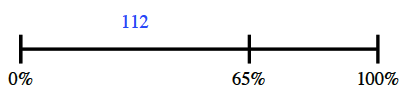### Home > CC1MN > Chapter 11 > Lesson 11.2.3 > Problem11-68

11-68.

Sixty-five percent of the 8th grade class is selling magazines for the class fundraiser. If $112$ students are selling magazines, how many students are in the 8th grade class?

$112$ students is $65\%$ of the class.
Draw a diagram like the one at right.

Now create a proportion equation that describes this relationship.

$x = 172.31$

Approximately $172$ students are in the 8th grade class.$\frac{65}{100}=\frac{112}x$